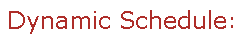Visit Minerva Class Schedule for course dates & times.

# MATH 423 Applied Regression (3 credits)

Offered by: Mathematics and Statistics (Faculty of Science)

### Overview

Mathematics & Statistics (Sci) : Multiple regression estimators and their properties. Hypothesis tests and confidence intervals. Analysis of variance. Prediction and prediction intervals. Model diagnostics. Model selection. Introduction to weighted least squares. Basic contingency table analysis. Introduction to logistic and Poisson regression. Applications to experimental and observational data.

Terms: Fall 2022

Instructors: Nadarajah, Tharshanna (Fall)